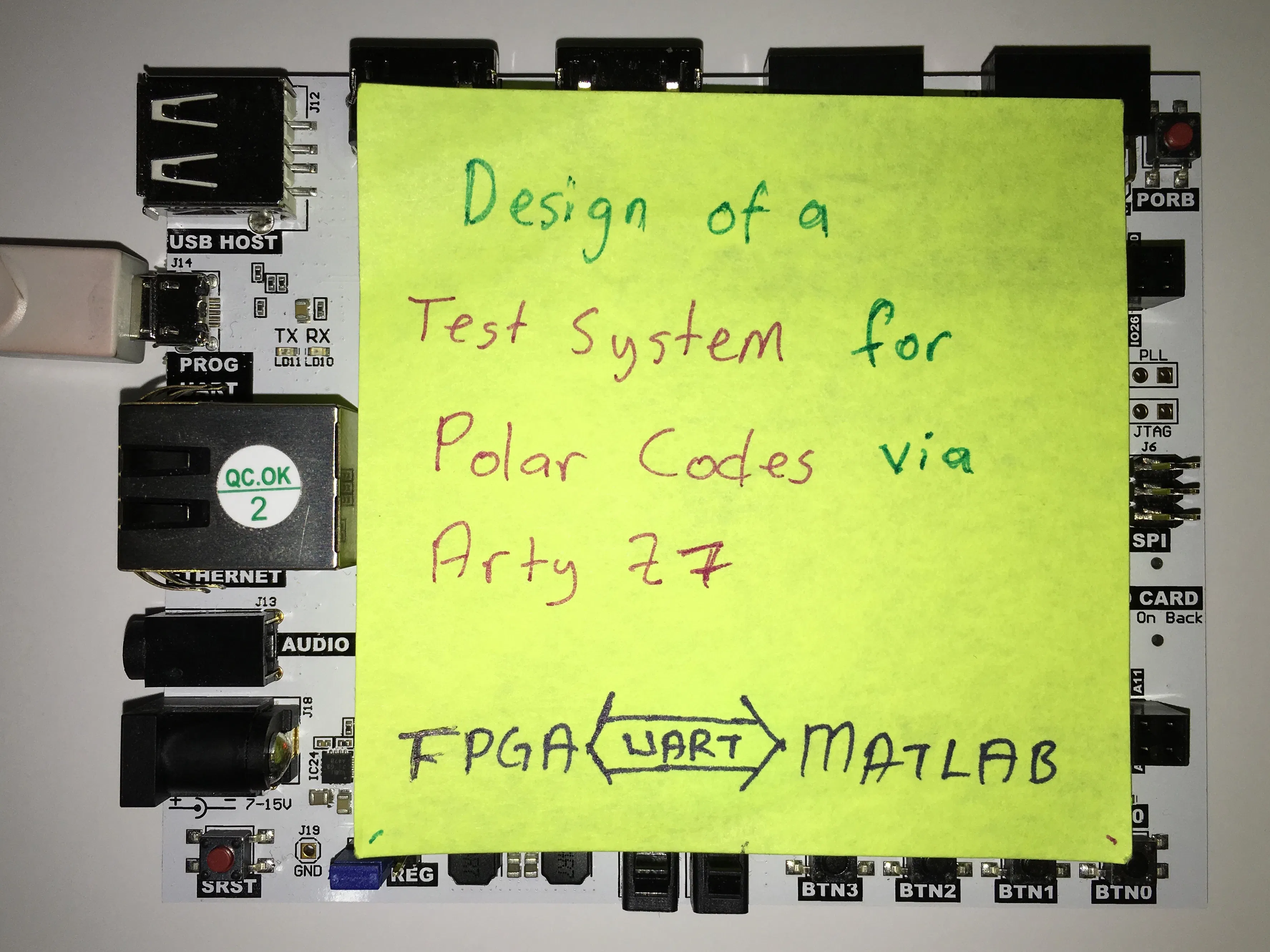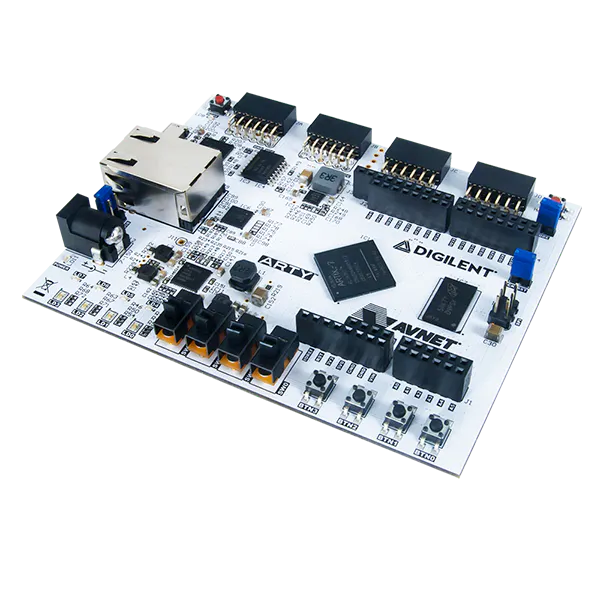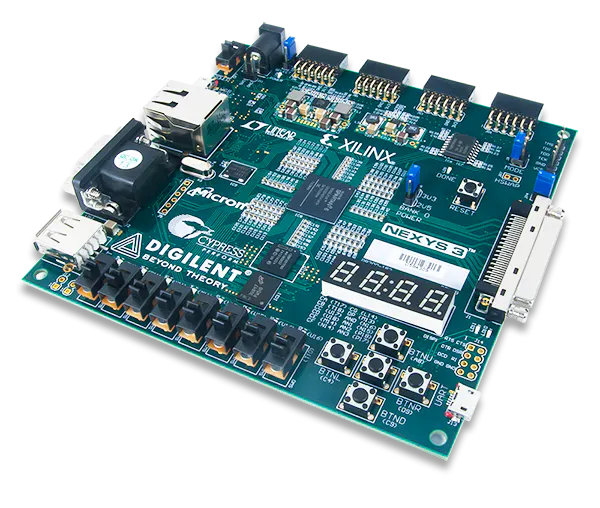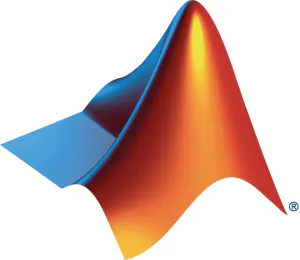# Design of a Test System for Polar Codes in FPGA

We design a flexible, remote-controlled test system for polar codes in FPGA.## Things used in this project

### Hardware componentsDigilent Arty A7-35T After the all tests successfully completed, we run our project in that board.
×1Digilent Nexys 3 Has more LEDs and switches, ideal for testing.
×1

### Software apps and online servicesMATLAB UART serial communication with FPGA for remote controlling, BER Calculations,
 AMD-Xilinx Vivado Writing and simulating VHDL codes for modern boards.
 Xilinx ISE Writing and simulating VHDL codes for Nexys family cards.
 Tera Term For easy UART testing.

## Code

VHDL
```library IEEE;
use IEEE.std_logic_1164.ALL;

entity main is
port(
clk: in std_logic;
reset,UART_RX,UART_TX_ENABLE: in bit;
ledout: out bit_vector (7 downto 0);
UART_LAST_TX: in bit_vector (7 downto 0);
UART_TX: out bit
);
end entity;

architecture logic_flow of main is
type UART_STATES is (IDLE,TX_START,BIT0,BIT1,BIT2,BIT3,BIT4,BIT5,BIT6,BIT7,STOP);
signal UART_STATE: UART_STATES := IDLE;
type UART_MODES is (RX,TX,IDLE);
signal UART_MODE: UART_MODES := IDLE;
signal UART_CLK: std_logic;
signal UART_CLK_COUNTER: natural range 0 to 5208:=0;
signal UART_LAST_RX: bit_vector (7 downto 0);
begin

process (reset, clk) begin
if (reset = '1') then
UART_CLK <= '0';
UART_CLK_COUNTER <= 0;
elsif rising_edge(clk) then
if(UART_CLK_COUNTER = 5208) then
UART_CLK <= NOT(UART_CLK);
UART_CLK_COUNTER <= 0;
else
UART_CLK_COUNTER <= UART_CLK_COUNTER + 1;
end if;
end if;
end process;

process(UART_CLK)
begin

if( reset='1') then
ledout <= "00000000";
UART_STATE <= IDLE;
UART_TX <= '1';
UART_MODE <= IDLE;
elsif( falling_edge(UART_CLK) ) then
case UART_STATE is
when IDLE =>
UART_MODE <= IDLE;
if (UART_RX='0') then
UART_MODE <= RX;
UART_STATE <= BIT0;
elsif (UART_TX_ENABLE='1') then
UART_MODE <= TX;
UART_STATE <= TX_START;
end if;
when TX_START =>
if(UART_MODE=TX) then
UART_TX <= '0';
end if;
UART_STATE <= BIT0;
when BIT0 =>
if(UART_MODE=RX) then UART_LAST_RX(0) <= UART_RX;
else UART_TX <= UART_LAST_TX(0); end if;
UART_STATE <= BIT1;
when BIT1 =>
if(UART_MODE=RX) then UART_LAST_RX(1) <= UART_RX;
else UART_TX <= UART_LAST_TX(1); end if;
UART_STATE <= BIT2;
when BIT2 =>
if(UART_MODE=RX) then UART_LAST_RX(2) <= UART_RX;
else UART_TX <= UART_LAST_TX(2); end if;
UART_STATE <= BIT3;
when BIT3 =>
if(UART_MODE=RX) then UART_LAST_RX(3) <= UART_RX;
else UART_TX <= UART_LAST_TX(3); end if;
UART_STATE <= BIT4;
when BIT4 =>
if(UART_MODE=RX) then UART_LAST_RX(4) <= UART_RX;
else UART_TX <= UART_LAST_TX(4); end if;
UART_STATE <= BIT5;
when BIT5 =>
if(UART_MODE=RX) then UART_LAST_RX(5) <= UART_RX;
else UART_TX <= UART_LAST_TX(5); end if;
UART_STATE <= BIT6;
when BIT6 =>
if(UART_MODE=RX) then UART_LAST_RX(6) <= UART_RX;
else UART_TX <= UART_LAST_TX(6); end if;
UART_STATE <= BIT7;
when BIT7 =>
if(UART_MODE=RX) then UART_LAST_RX(7) <= UART_RX;
else UART_TX <= UART_LAST_TX(7); end if;
UART_STATE <= STOP;
when STOP =>
if(UART_MODE=RX) then
if (UART_RX='1') then
UART_STATE <= IDLE;
ledout <= UART_LAST_RX;
else
UART_STATE <= BIT0;
end if;
else
UART_TX <= '1';
UART_STATE <= IDLE;
end if;
end case;
end if;
end process;

end;
```

### Polar Encoder

VHDL
```library IEEE;
use IEEE.STD_LOGIC_1164.ALL;

entity polar_encoder is
generic(

TOTAL_BITS:NATURAL:=8;
TOTAL_BITS_LOG2:NATURAL:=3

);
port(clk:in std_logic;
y0:out bit_vector(1 to TOTAL_BITS);
y1:out bit_vector(1 to TOTAL_BITS);
y2:out bit_vector(1 to TOTAL_BITS);
y3:out bit_vector(1 to TOTAL_BITS);
y4:out bit_vector(1 to TOTAL_BITS);
y5:out bit_vector(1 to TOTAL_BITS);
y6:out bit_vector(1 to TOTAL_BITS);
y7:out bit_vector(1 to TOTAL_BITS);
encoded:out bit_vector(1 to TOTAL_BITS)
);
end entity;

architecture logic_flow of polar_encoder is
type bit_vector_2d is array(1 to TOTAL_BITS) of bit_vector(1 to TOTAL_BITS);
signal G_MATRIX : bit_vector_2d;
signal generate_G_MATRIX: std_logic;
signal generated_G_MATRIX: std_logic;
signal ZERO_ROW:bit_vector(1 to TOTAL_BITS) := (others => '0');
signal to_be_encoded: bit_vector(1 to TOTAL_BITS);

----------------------
function POLAR_ENCODE( MESSAGE : in bit_vector; G_MATRIX : in bit_vector_2d )
return bit_vector is
variable ENCODED : bit_vector(1 to TOTAL_BITS);
variable XOR_temp : bit := '0';
begin

for I in 1 to TOTAL_BITS loop
XOR_temp := '0';
for J in 1 to TOTAL_BITS loop
if( G_MATRIX(J)(I) = '1' ) then
XOR_temp := XOR_temp XOR MESSAGE(J);
end if;
end loop;
ENCODED(I) := XOR_temp;
end loop;

return ENCODED;

end function POLAR_ENCODE;
------------------------

begin

generate_G_MATRIX <= '1';

y0 <= G_MATRIX(1);
y1 <= G_MATRIX(2);
y2 <= G_MATRIX(3);
y3 <= G_MATRIX(4);
y4 <= G_MATRIX(5);
y5 <= G_MATRIX(6);
y6 <= G_MATRIX(7);
y7 <= G_MATRIX(8);

G_MATRIX_GENERATOR: process(generate_G_MATRIX)
variable current_dimension: positive:=2;
variable next_g_matrix,current_g_matrix: bit_vector_2d;
begin
if(current_dimension=2) then
--current_g_matrix:=(others => ZERO_ROW);
current_g_matrix(1)(1 to 2) := "10";
current_g_matrix(2)(1 to 2) := "11";
for I in 1 to TOTAL_BITS_LOG2-1 loop
next_g_matrix:=(others => ZERO_ROW);
for J in 1 to current_dimension loop
for K in 1 to current_dimension loop
next_g_matrix(K)(2*J-1):= current_g_matrix(K)(J);
next_g_matrix(K+current_dimension)(2*J-1):= current_g_matrix(K)(J);
next_g_matrix(K+current_dimension)(2*J):= current_g_matrix(K)(J);
end loop;
end loop;
current_g_matrix := next_g_matrix;
current_dimension:=current_dimension*2;
end loop;
G_MATRIX<=next_g_matrix;
end if;
end process;

to_be_encoded <= "00010101";
encoded <= POLAR_ENCODE(to_be_encoded,G_MATRIX);

end architecture;
```

MATLAB
For receive data, first version of code.
```%% uart connect
s = serial('COM6');
set(s,'BaudRate',9600);
fopen(s);

i = 0;
while i<10000

if(length_of_binary<8)
end
i=i+1;

end

%% clear
fclose(s);delete(s);clear s
```

### UART Transmitter MATLAB

MATLAB
```%% uart connect
s = serial('COM6');
set(s,'BaudRate',9600);
fopen(s);

%% UART transmitter
command_to_send_vector = [0 0 0 1 0 0 0 1];
command_to_send = char(command_to_send_vector + '0');
command_to_send_ASCII = bin2dec(command_to_send);
fwrite(s,command_to_send_ASCII,'char')
%% clear
fclose(s);delete(s);clear s
```

## Credits

### halukunal

1 project • 1 follower

### omeruzunoglan

1 project • 2 followers